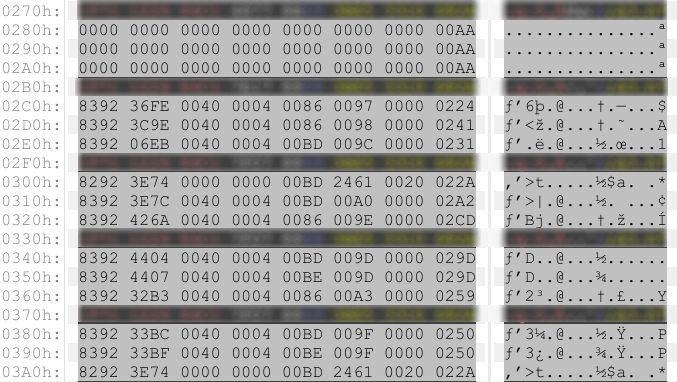# Cracking a MAC used in Mifare Cards

Sep 22, 18When working on NFC systems it is common to find MACs used to prevent users from fiddling with the data stored in the chip.

Still, it’s good fun to fiddle with data. Let’s see if we can figure out a MAC used in a real world application.

# Working out the MACMAC collisions

We can be quite sure this is not a complex algo simply looking at a single (albeit lucky) dump. There are many collisions (highlighted in different colors).

Given the first 3 sectors are all zeroed and still we have `AA` as the checksum we could think to a final sum or xor. Sums might overflow, though, then xor is our favorite contender. We now have the probable “final xor” (as it is called in CRC)

But what now?

Since we’re using a xor (probably) as a final operation, it might be used elsewhere. `00 xor 00` is 00, actually, which goes along with our first sectors.

The collisions can help us now. What’s different among the two lines with `50` as a CRC? Odd bytes stay the same, while even bytes change: `BC` becomes `BF` and `BD` is `BE`. What if we XOR these 2 values together? `BC xor BD = 1` and `BF xor BE = 1`, too, and the same goes for the other relevant collision. This is good, when there is a collision, the even bytes xorred together give a constant. This might be the right track.

It’s now quite obvious how this MAC could possibly be generated. You have to xor together odd bytes, then do the same for even bytes. What if we finally xor these 2 together and finally xor the result with AA (we know this thanks to the first line)?

``````83 xor 33 xor 00 xor 00 xor 00 xor 00 xor 00 xor 02 = B2
92 xor BC xor 40 xor 04 xor BD xor 9F xor 00 = 48
B2 xor 48 = FA
FA xor AA = 50
``````

BINGO!

# Python to the rescue

Using your system’s calculator is fun and all, but as humans we’re prone to errors (I am especially good at it), you don’t want your test to fail because you put somewhere a byte wrongly. Python does not fail (at least in this case)

``````#!/usr/bin/python3
import sys

if (len (sys.argv) is not 2):
print ("Missing input string or too many args")
print ("CRC.py <15 bytes hex string>")
exit (1)

input_trimmed = sys.argv.replace(" ", "")
if (len(input_trimmed) is not 30):
print ("Hex string is not 15 bytes long")
exit (1)

even_bytes = 0x00
odd_bytes = 0x00
for i in range (2, 27, 4):
even_bytes ^= int(input_trimmed[i:i+2], 16)
for i in range (0, 30, 4):
odd_bytes ^= int(input_trimmed[i:i+2], 16)
print ("Even bytes: " + hex(even_bytes) + "\t\tOdd bytes: " + hex(odd_bytes))

xor_even_odd = even_bytes ^ odd_bytes
MAC = xor_even_odd ^ 0xaa

print ("MAC:\t" + hex(MAC))
``````

# Conclusion

This was a quick one, I’m not even sure it was worth blogging, but I thought this site was dying and wanted to do something about it…